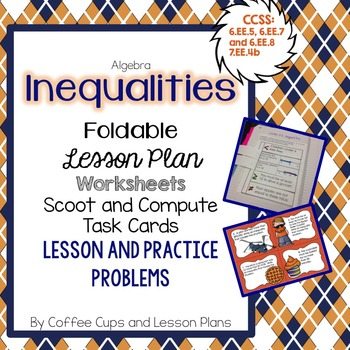# Inequalities Foldable for Interactive Notebook with Lesson Plan & Worksheets6th - 7th, Homeschool
Subjects
Standards
Resource Type
Formats Included
• Zip
Pages
26 pages

### Description

Set contains:
Foldie and Lesson plan for Interactive Notebook,
4 worksheets for practice in class or homework
1 Blank worksheet to create your own inequalities to graph OR graph a set of inequalities and have students identify.
16 Scoot and Compute Task Cards

*** PLEASE NOTE-- TASK CARDS ARE ALSO SOLD SEPARATELY*** THESE ARE THE SAME CARDS.
Cards contain both computation and word problems.

CCSS: 6.EE5, 6.EE.7, 6.EE.8 and 7.EE.4b
Total Pages
26 pages
Included
Teaching Duration
N/A
Report this Resource to TpT
Reported resources will be reviewed by our team. Report this resource to let us know if this resource violates TpT’s content guidelines.

### Standards

to see state-specific standards (only available in the US).
Solve word problems leading to inequalities of the form 𝘱𝘹 + 𝘲 > 𝘳 or 𝘱𝘹 + 𝘲 < 𝘳, where 𝘱, 𝘲, and 𝘳 are specific rational numbers. Graph the solution set of the inequality and interpret it in the context of the problem. For example: As a salesperson, you are paid \$50 per week plus \$3 per sale. This week you want your pay to be at least \$100. Write an inequality for the number of sales you need to make, and describe the solutions.
Write an inequality of the form 𝘹 > 𝘤 or 𝘹 < 𝘤 to represent a constraint or condition in a real-world or mathematical problem. Recognize that inequalities of the form 𝘹 > 𝘤 or 𝘹 < 𝘤 have infinitely many solutions; represent solutions of such inequalities on number line diagrams.
Solve real-world and mathematical problems by writing and solving equations of the form 𝘹 + 𝘱 = 𝘲 and 𝘱𝘹 = 𝘲 for cases in which 𝘱, 𝘲 and 𝘹 are all nonnegative rational numbers.
Understand solving an equation or inequality as a process of answering a question: which values from a specified set, if any, make the equation or inequality true? Use substitution to determine whether a given number in a specified set makes an equation or inequality true.